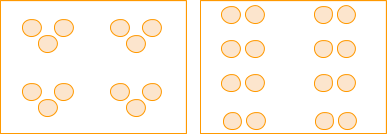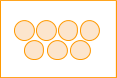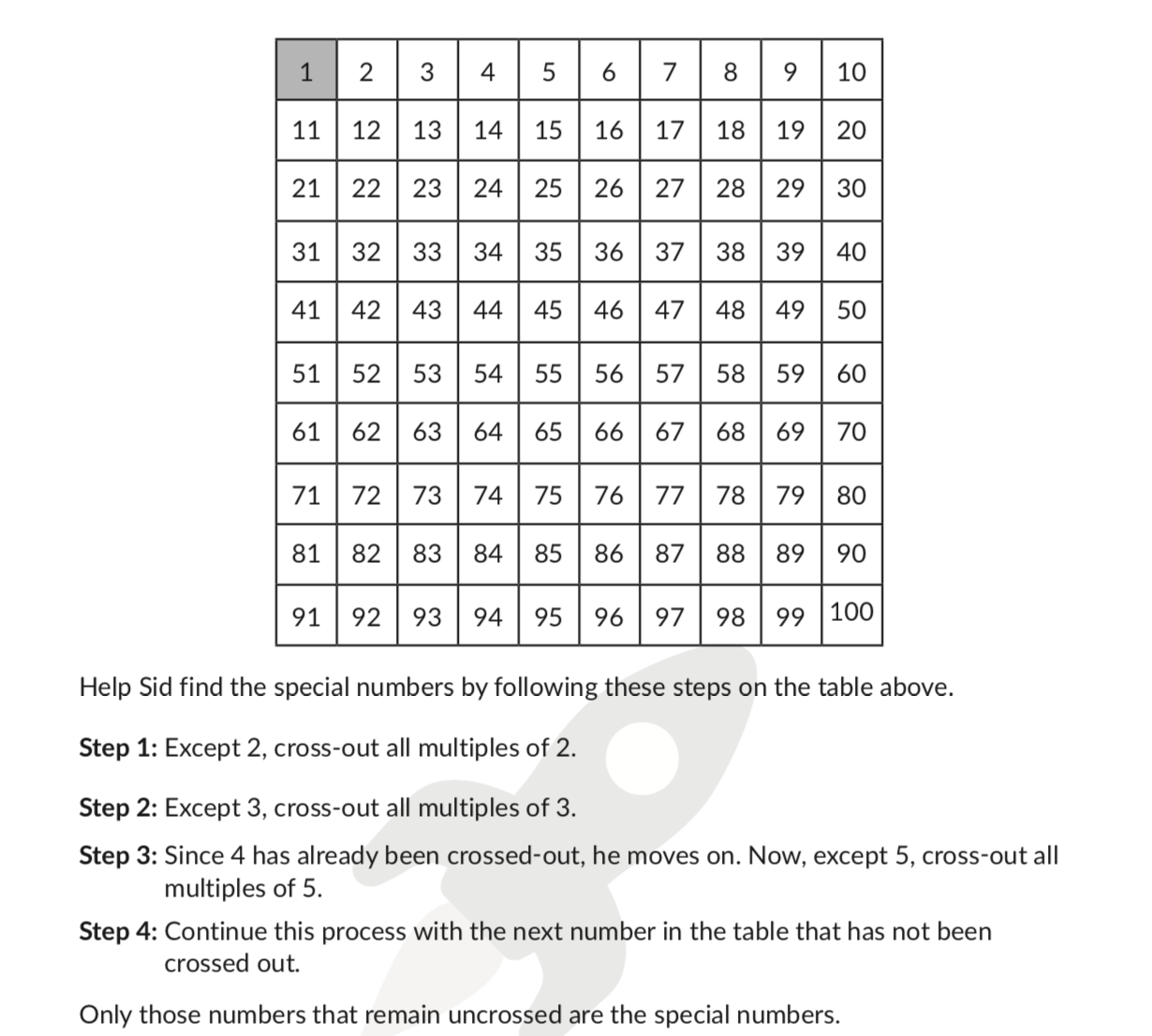# Prime Numbers

Go back to  'Factors, Multiples, Primes'

## An easy way to understand Prime Numbers

If a number can be divided into equal groups, then it is not a Prime number. It is a Composite number.

If a number cannot be divided into equal groups, then it is a Prime number.
Keep in mind:

• groups cannot be of size one.
• groups cannot have fractional quantities like $$\frac{1}{2}$$ in each group.

Let’s take a few examples: 12 can be divided into:• 2 groups of 6
• 3 groups of 4
• 4 groups of 3
• 6 groups of 2
• (1 group of 12 or 12 groups of 1 don't count in our current system)

Hence 12 is not a Prime number. 12 is a composite number.

Quick recall: 1, 2, 3, 4, 6 and 12 are called factors or 12

Another example: 7 cannot be divided into groups of 2 or 3 or 4 or any other whole number (remember 7 groups of 1 or 1 group of 7 doesn't count). So, 7 is a Prime number.Quick recall: 1 and 7 are the only factors of 7.

### An Easy Definition of Prime Numbers

 Any whole number greater than 1 that has exactly two factors, 1 and itself, is defined to be a prime number.

Another equivalent definition is

 Any whole number greater than 1 that is divisible only by 1 and itself, is defined as a prime number.

Any number greater than 1 that is not a Prime number, is defined to be a composite number. Thus composite numbers will always have more than 2 factors.

1 is neither prime nor composite. See the reason for it below.

Any whole number greater than 1 is either a Prime or Composite.

For example, the number 15 has more than two factors: 1, 3, 5, and 15. Hence it is a composite number. On the other hand, 13 has just two factors: 1 and 13. Hence it is a prime number.

### Examples of Prime Numbers and Composite Numbers

Here are some examples of Prime and Composite numbers with the reasoning behind that label. Using this reasoning you can find out whether any given number is prime or composite.

1. Is 4 a Prime number?
• How many factors does 4 have?
• Factors of 4 are: 1, 2 and 4.
• Since 4 has more than two factors, it is not a prime number. It is a composite number.
1. Is 7 a Prime number?
• How many factors does 7 have?
• Factors of 7 are: 1 and 7.
• 7 has just two factors: 1 and itself. Hence it is a prime number.
1. Is 13 a Prime number?
• How many factors does 13 have?
• Factors of 13 are: 1 and 13.
• 13 has just two factors: 1 and itself. Hence it is a prime number.
1. Is 20 a Prime number?
• How many factors does 20 have?
• Factors of 20 are: 1, 2, 4, 5, 10 and 20.
• Since 20 has more than two factors, it is not a prime number. It is a composite number.

## How to find out if a Number is a Prime Number?

Math is best learnt through activities. Here’s a beautiful activity that helps you find out if a number is prime or composite. This activity works best for numbers between 2 to 100.

### Activity to find out all Prime Numbers till 100

Start with a grid with numbers listed from 1 to 100. We cross out 1 because Prime numbers are defined for numbers greater than 1.• Step 1: We have crossed out 1. So we start with the next available number. That’s 2. Circle it. This is your first prime number.

• Step 2: Now cross out all multiples of 2. Continue until you reach the end of the grid. So almost half the number up to 100 will get crossed out. These cannot be prime as they have 2 as their factor apart from 1 and themselves. So all multiples are composite numbers.

• Step 3: Move to the next available number. That will be 3. Circe it. You’ve found another prime number!

• Step 4: Cross out all multiples of 3. Continue until you reach the end of the grid. If a number is already crossed out, e.g. 6 just move on. The last number you’ll cross out will be 99.

• Step 5: Move to the next available number. This will be 5. Circle it. You’ve found another prime number!

• Step 6: Cross out all multiples of 5.

• Step 7: Move to the next available number and circle it. Then cross out its multiples. Repeat step 7 till all numbers on the grid are either crossed or circled.

The numbers you circled are Prime Numbers. The numbers you crossed are composite numbers.

### Another quick way to find if a number is a prime number or not

Is 97 a prime number?

1. We use divisibility rules to quickly find out if 97 is divisible by 2, 3, 5.
• 97 is an odd number - so not divisible by 2.
• The digits of 97 add up to 16 which is not divisible by 3. So 97 is not divisible by 3.
• Since the units place digit is not 0 or 5, 97 is not divisible by 5.
• We can skip 4, 6, 8, 9, 10, 12 and 15 as any number divisible by these would have also been divisible by 2 or 3 or 5.
1. Now let’s check some of the subsequent numbers like 7 and 11. Using whichever technique including long division we see that 97 is not divisible by any of these numbers.
• Also, observe that 11 x 9 = 99, a number greater than 97. That means we’ve gone past the square root of 97. This gives us an important clue. If you’ve gone past the square root of the given number without finding any factors, then you need not continue. You won't find any new factors.
• The reason for this is for all subsequent numbers (larger than 11), the multiplicand will be smaller than 9. Since we’ve checked all numbers till 9 (in fact till 11 already), we know that no pair of factors is possible. Remember all factors pairs will have one number smaller than the square root and the other larger than the square root. (A factor pair is a set of two numbers which when multiplied give you the original number whose factors you’re identifying).
1. Thus 97 does not have any factors apart from 1 and 97. So, 97 is a prime number.

### Why are Prime Numbers Important?

Prime numbers can be considered the building blocks of numbers. Any whole number is either a prime number or can be built by multiplying a unique combination of prime numbers.

That means all composite numbers have a recipe to be built using prime numbers.

Let’s consider a few composite numbers:

• 4 is a composite number.
It can be built by multiplying the prime number 2 twice.
2 x 2 = 4
• 12 is a composite number.
It can be built by multiplying the prime numbers 2 and 3 is a certain combination.
2 x 2 x 3 = 12
• 105 is a composite number.
It can be built by multiplying the prime number 3, 5 and 7.
3 x 5 x 7 = 105

In this way, any whole number you pick will either be a prime number itself or can be built using a unique combination of prime numbers.

This is a very important idea in mathematics and is called the Fundamental Theorem of Arithmetic.

Hence prime numbers are sometimes called the building blocks of all numbers.

### Why is 1 not a Prime Number?

There are many ways to think about this. Prime numbers are most effective because using them we can create a unique combination for any composite number.
E.g. the composite number 147 is built using 3 x 7 x 7.

If we consider 1 to be a prime number, the combination of prime numbers that create 147 will no longer remain unique:

• Combination 1: 147 = 3 x 7 x 7 x 1
• Combination 2: 147 = 3 x 7 x 7 x 1 x 1

Etc.

This is not very practical. Hence the definition of prime numbers excludes 1.

### What are twin Primes?

A pair of Prime Numbers that differs by 2 are called twin primes.
E.g: 3 and 5 are twin primes. 5 and 7 are twin primes. 11 and 13 is another pair of twin primes.

### What are co Primes?

If a pair of numbers has no common factor apart from 1, then they are called co-prime numbers.
E.g. 8 and 21 are co-primes. That’s because factors of 8 are 1, 2, 4 and 8. Factors of 21 are 1, 3, 7 and 21. So 8 and 21 have no common factors apart from 1, making them co-prime.
List of all prime numbers between 1 to 100
There are 25 prime numbers till 100. They are: 2, 3, 5, 7, 11, 13, 17, 19, 23, 29, 31, 37, 41, 43, 47, 53, 59, 61, 67, 71, 73, 79, 83, 89, 97.

 Prime numbers between 1 to 10 2, 3, 5, 7 Prime numbers between 11 to 20 11, 13, 17, 19 Prime numbers between 21 to 30 23, 29 Prime numbers between 31 to 40 31, 37 Prime numbers between 41 to 50 41, 43, 47 Prime numbers between 51 to 100 53, 59, 61, 67, 71, 73, 79, 83, 89, 97

### All prime numbers between 1 and 200

There are 46 prime numbers till 200. They are 2, 3, 5, 7, 11, 13, 17, 19, 23, 29, 31, 37, 41, 43, 47, 53, 59, 61, 67, 71, 73, 79, 83, 89, 97, 101, 103, 107, 109, 113, 127, 131, 137, 139, 149, 151, 157, 163, 167, 173, 179, 181, 191, 193, 197, and 199.

## Tips and Tricks to Identify Prime Numbers

• An even number greater than 2 will always be composite. 2 is the only even Prime number.
• If a number with 2 or more digits ends with 5 or 0 it cannot be a prime number. That’s because such numbers will always have 5 as a factor.

### Common mistakes or misconceptions related to Prime numbers

• Misconception: 1 is a Prime number
The definition of Prime numbers applies for numbers greater than 1. So 1 is not included. It is neither Prime nor Composite.
• Misconception: Many other numbers can also be neither prime, not composite
All whole numbers greater than 1 are either Prime or Composite.
• What about fractions, decimals, rational numbers, and integers? Can they also be Prime numbers?
The definition of Prime numbers is based on whole numbers greater than 1. So fractions, decimals, rational numbers, that are not whole numbers are not considered to be Prime or Composite. Negative integers get eliminated as they are not greater than 1.

1. Is 53 a Prime number?
____________________

2. Is 72 a Prime number?
____________________

3. How many factors does 15 have?
____________________

4. Is \begin{align} \frac{3}{4} \end{align} a Prime number?
____________________

5. How many Prime Numbers are there between 60 and 70?
____________________